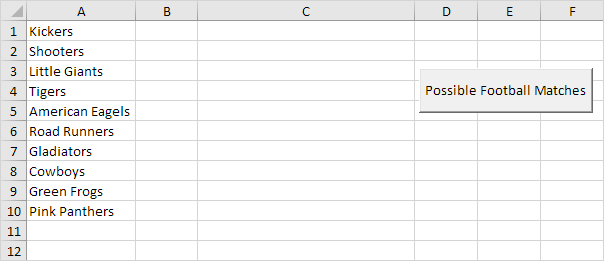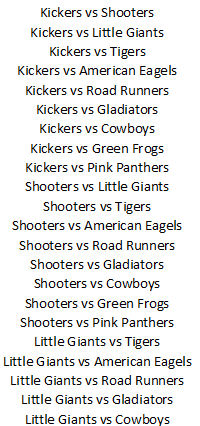# Possible Football Matches

Below we will look at a program in Excel VBA that shows a print preview of all the possible football matches from a list of teams.

Situation:1. First, we declare one Range object and four variables. We call the Range object rng. One String variable we call matchname, and three Integer variables we call counter, i and j.

Dim rng As Range, matchname As String, counter As Integer, i As Integer, j As Integer

2. We initialize rng with the team names. We use CurrentRegion because we don't know the exact boundaries of the range in advance (we want this program to work for 3 teams but also for 12 teams). We initialize counter with value 0.

Set rng = Range("A1").CurrentRegion
counter = 0

3. We write all the possible football matches to column C. First, we empty column C.

Worksheets(1).Columns(3) = ""

4. We start a Double Loop.

For i = 1 To rng.Count
For j = i + 1 To rng.Count

5. We write a matchname to the variable matchname.

matchname = rng.Cells(i).Value & " vs " & rng.Cells(j).Value

For example, for i = 1 and j = 2, Excel VBA writes the matchname Kickers vs Shooters. For i = 1 and j = 3, Excel VBA writes the matchname Kickers vs Little Giants, etc.

6. We write the matchname to column C.

Cells(counter + 1, 3).Value = matchname

7. The counter keeps track of the number of matchnames written to column C. Excel VBA increments counter by 1 each time it writes a matchname to column C. To achieve this, add the following code line:

counter = counter + 1

8. Don't forget to close the two loops.

Next j
Next i

9. We show a print preview of all the possible football matches.

ActiveSheet.Columns(3).PrintPreview

10. Test the program.

Part of the result:Note: column C is manually centered to get this result.

Go to Next Chapter: Variables